Chapter 14 Class 10 Statistics

Class 10
Important Questions for Exam - Class 10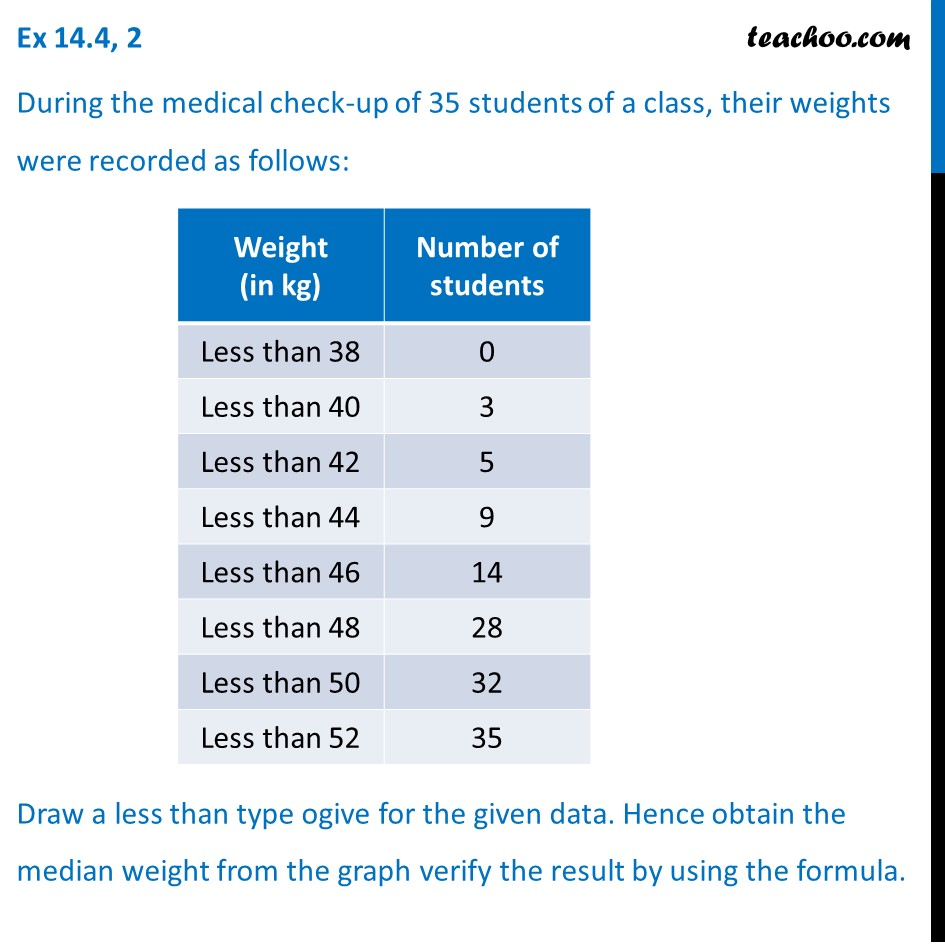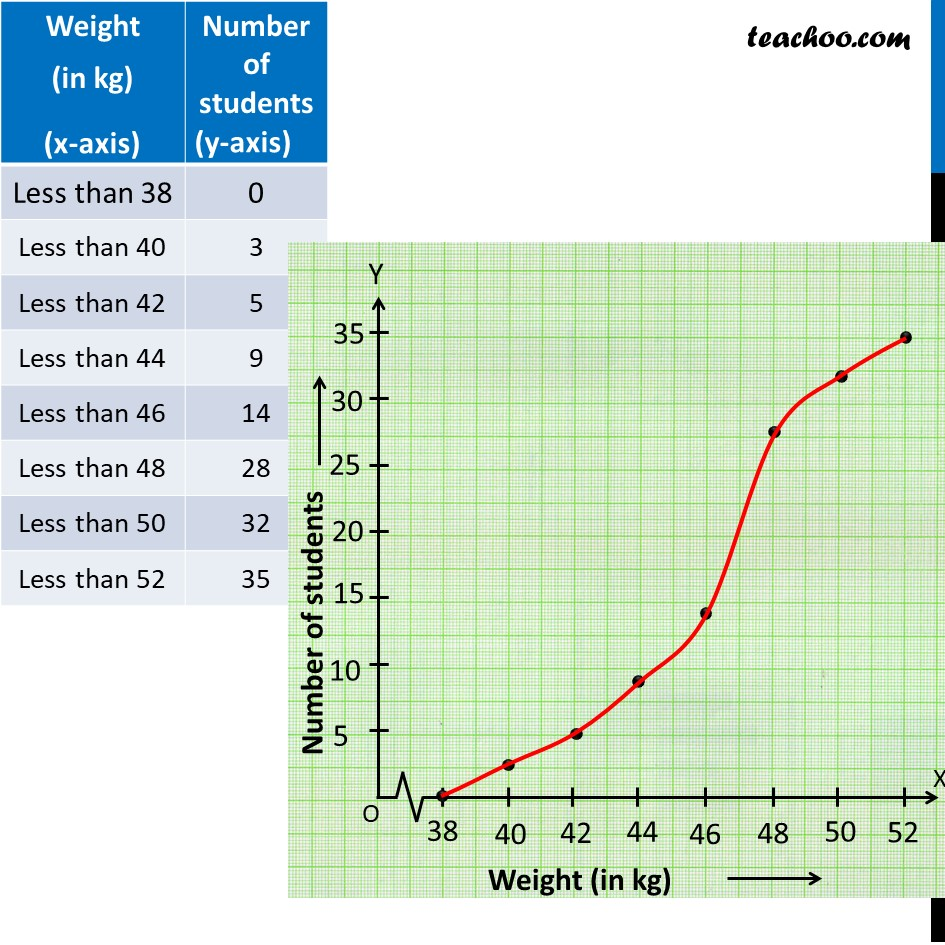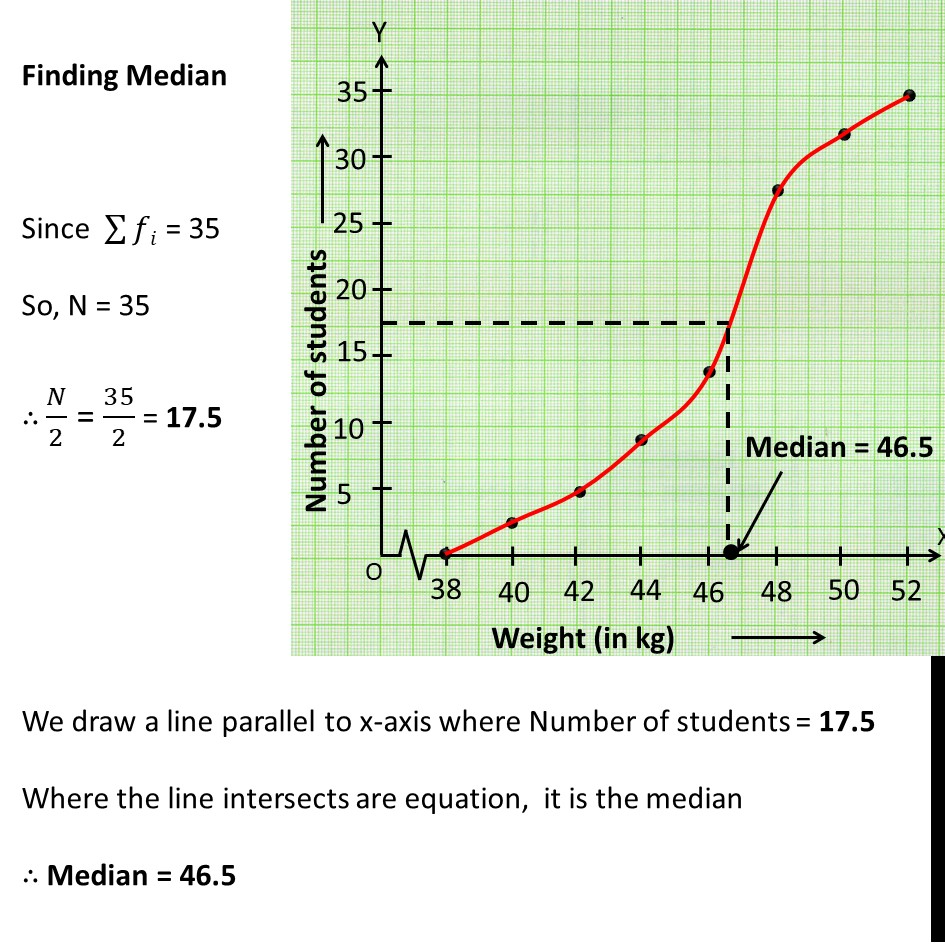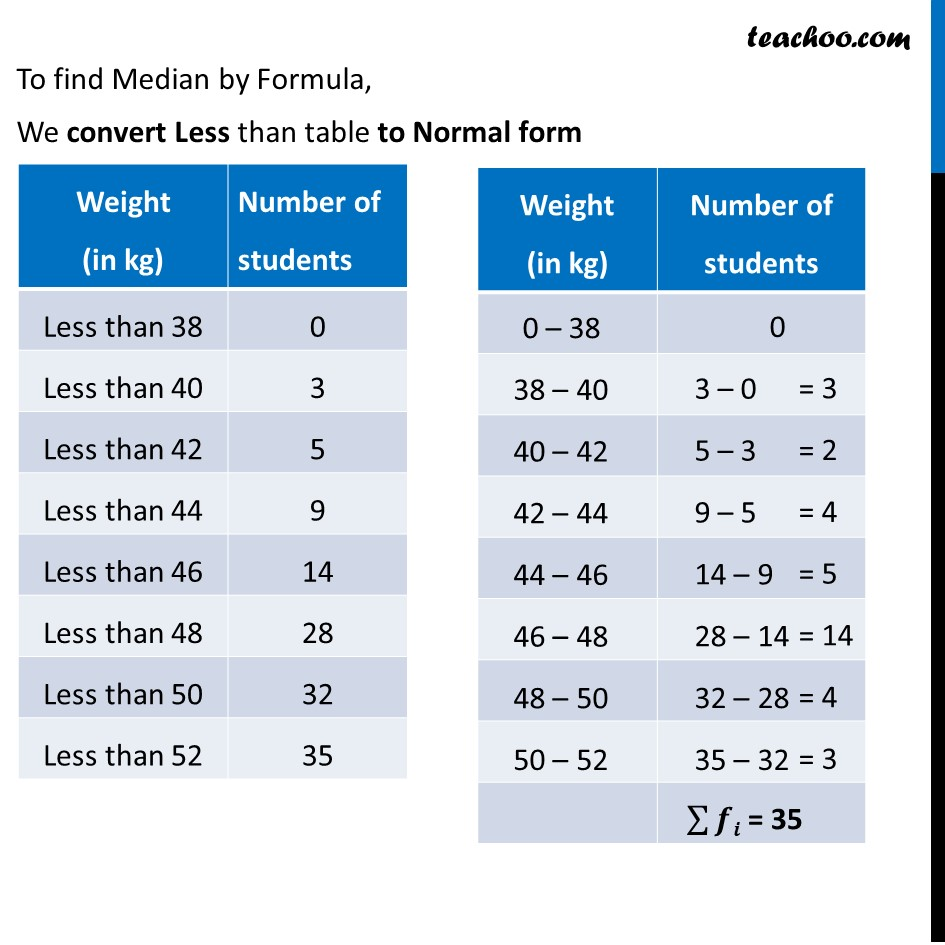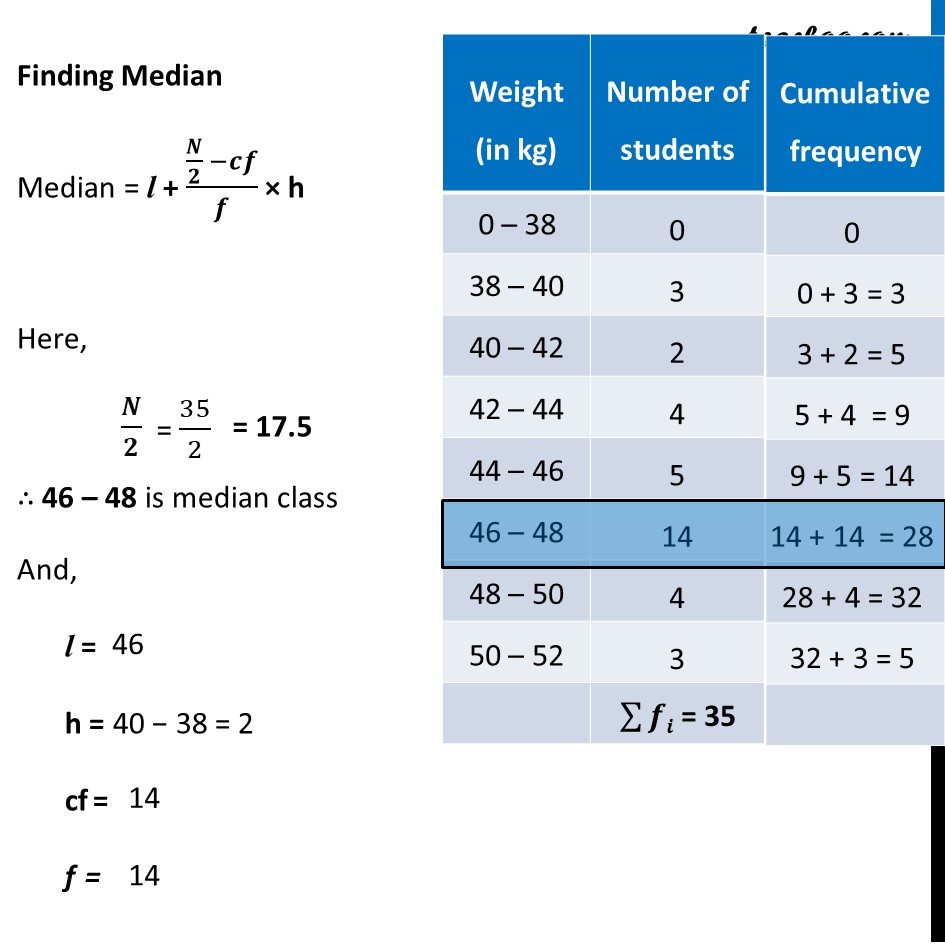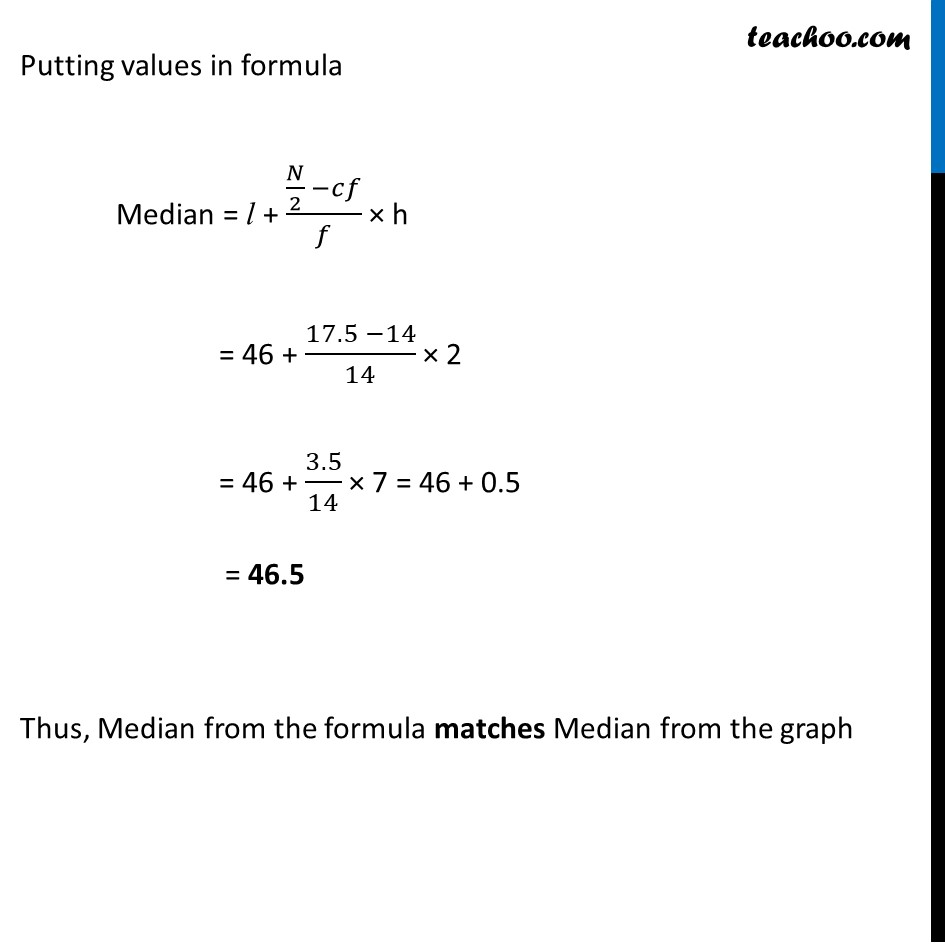Learn in your speed, with individual attention - Teachoo Maths 1-on-1 Class

### Transcript

Question 2 During the medical check-up of 35 students of a class, their weights were recorded as follows: Draw a less than type ogive for the given data. Hence obtain the median weight from the graph verify the result by using the formula. Finding Median Since ∑▒𝑓𝑖 = 35 So, N = 35 ∴ 𝑁/2 = 35/2 = 17.5 We draw a line parallel to x-axis where Number of students = 17.5 Where the line intersects are equation, it is the median ∴ Median = 46.5 To find Median by Formula, We convert Less than table to Normal forFinding Median Median = l + (𝑵/𝟐 −𝒄𝒇)/𝒇 × h Here, 𝑵/𝟐 ∴ 46 – 48 is median class And, l = h = cf = f = Putting values in formula Median = l + (𝑁/2 −𝑐𝑓)/𝑓 × h = 46 + (17.5 −14)/14 × 2 = 46 + 3.5/14 × 7 = 46 + 0.5 = 46.5 Thus, Median from the formula matches Median from the graph